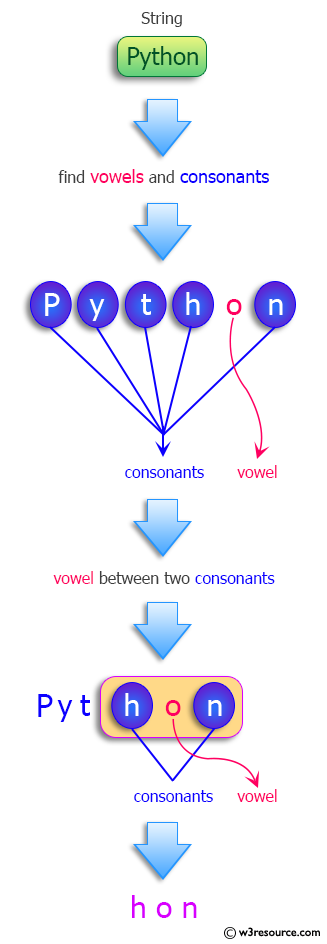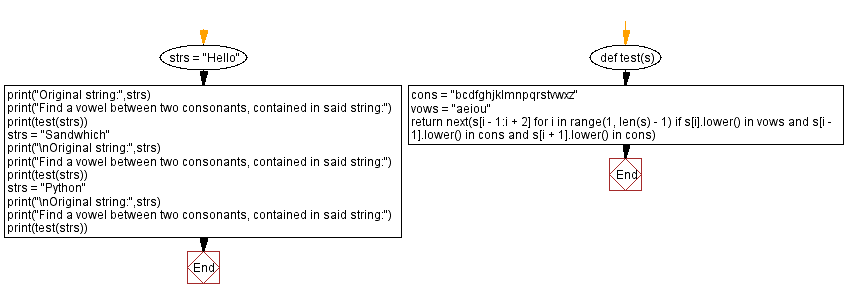﻿ Python: Find a string contains a vowel between two consonants, in a given string - w3resource# Python: Find a string contains a vowel between two consonants, in a given string

## Python Programming Puzzles: Exercise-73 with Solution

Write a Python program to find a substring in a given string contains a vowel between two consonants.

From vocabulary.com:
A consonant is a speech sound that is not a vowel. It also refers to letters of the alphabet that represent those sounds: Z, B, T, G, and H are all consonants. Consonants are all the non-vowel sounds, or their corresponding letters: A, E, I, O, U and sometimes Y are not consonants.

```Input: Hello
Output:
Hel

Input: Sandwhich
Output:
San

Input: Python
Output:
hon
```

Pictorial Presentation:Sample Solution:

Python Code:

``````#License: https://bit.ly/3oLErEI

def test(s):
cons = "bcdfghjklmnpqrstvwxz"
vows = "aeiou"
return next(s[i - 1:i + 2] for i in range(1, len(s) - 1)
if s[i].lower() in vows and s[i - 1].lower() in cons and s[i + 1].lower() in cons)
strs = "Hello"
print("Original string:",strs)
print("Find a vowel between two consonants, contained in said string:")
print(test(strs))
strs = "Sandwhich"
print("\nOriginal string:",strs)
print("Find a vowel between two consonants, contained in said string:")
print(test(strs))
strs = "Python"
print("\nOriginal string:",strs)
print("Find a vowel between two consonants, contained in said string:")
print(test(strs))
``````

Sample Output:

```Original string: Hello
Find a vowel between two consonants, contained in said string:
Hel

Original string: Sandwhich
Find a vowel between two consonants, contained in said string:
San

Original string: Python
Find a vowel between two consonants, contained in said string:
hon
```

Flowchart:## Visualize Python code execution:

The following tool visualize what the computer is doing step-by-step as it executes the said program:

Python Code Editor :

Have another way to solve this solution? Contribute your code (and comments) through Disqus.

What is the difficulty level of this exercise?

Test your Programming skills with w3resource's quiz.

﻿

## Python: Tips of the Day

Clamps num within the inclusive range specified by the boundary values x and y:

Example:

```def tips_clamp_num(num,x,y):
return max(min(num, max(x, y)), min(x, y))
print(tips_clamp_num(2, 4, 6))
print(tips_clamp_num(1, -1, -6))
```

Output:

```4
-1
```